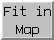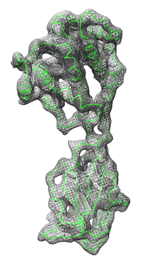### Fit in MapFit in Map locally optimizes the fit of atomic coordinates into a density map or one density map into another. The maps usually represent electron density, but other types of volume data can also be used. See also: Volume Viewer, SegFit, Values at Atom Positions, measure, molmap, sym, saving maps after fitting

There are several ways to start Fit in Map, a tool in the Volume Data category (including from the Volume Viewer Tools menu). It is also implemented as the command fitmap. However, the command provides some notable features that are not available in Fit in Map:

The atomic structures and/or map(s) of interest should first be opened in Chimera. The fit model (atoms or map to fit) and reference map can then be chosen from the respective menus in the Fit in Map dialog. Since the optimization is local rather than global, the fit model should be placed in a trial position relative to the reference map before fitting. This usually involves interactive manipulation and toggling models between active and immovable states. When atoms are fit, an entire molecule model or just the currently selected atoms can be used.

Clicking Fit performs local optimization of the fit (atoms-in-map or map-in-map) using the current options. The calculation will stop and the fit model will be repositioned after the earliest of:

Clicking Fit again may further improve the results, especially if convergence was not reached. Undo backtracks through transformations of the fit model relative to the reference map applied by Fit in Map, whereas Redo reapplies them.

Find Clashes/Contacts can be used to monitor for close contacts between atomic structures as they are moved, and atomic coordinates can be saved relative to the reference map.

Clicking Update gives the current atoms-in-map and/or map-in-map fit values without performing any fitting.

Clicking Results opens the Reply Log, which along with the fit values reports the number of steps taken and fit model displacement (translational shift and angle of rotation) since Fit was clicked. The transformation of the fit model relative to the reference map is described with a transformation matrix and as an axis of rotation (a unit vector), point on the axis, degrees of rotation, and shift parallel to the axis.

Clicking Options reveals additional settings (clicking the close buttonon the right hides them again):

• Real-time correlation / average update - update fit values continuously as a model is moved
• Use map simulated from atoms, resolution [r] - generate a density map from the fit model atomic coordinates and use map-in-map fitting (but report both atoms-in-map and map-in-map fit values). The map is generated by describing each atom as a Gaussian distribution of width proportional to r and amplitude proportional to the atomic number (other parameters set to molmap defaults).
• Use only data above contour level from first map - exclude data outside the fit map contour surface from map-in-map fitting
• whether to Optimize the overlap or the correlation during map-in-map fitting
• Correlation calculated about mean data value - whether the mean values or zero (default) should be used to calculate the correlation during map-in-map fitting
• whether to Allow fit model rotation and/or shift movements
• Move whole molecules - when fitting selected atoms, whether to move just those atoms or the entire molecule model(s) containing them. Regardless of this setting, only the selected atoms will be used to calculate the fit.

Atoms-in-map fitting:
The average map value at fit atom positions is maximized. For each atom within the bounds of the reference map, the map value is found by trilinear interpolation from the eight corners of the enclosing data grid cell. Atoms outside the bounds of the map are not used for computing averages.
Map-in-map fitting:
Either the overlap or the correlation can be maximized. The calculation can include all nonzero-valued fit map grid points or only those with values above the map's lowest contour level in Volume Viewer (see options). The overlap is the sum over fit map grid points of the product of the fit map value and the reference map value at that point, determined by trilinear interpolation. It can be expressed as the inner product of vectors u and v containing the fit map values and the corresponding interpolated reference map values:
overlap = <u,v>
In similar notation,
 correlation = | u || v |
or if the correlation about mean option is turned on,
 correlation = |u–uave||v–vave|
where uave is a vector with all components equal to the average of the components of u and vave is defined analogously. The correlation equals the cosine of the angle between the vectors (after subtraction of averages) and can range from –1 to 1, whereas the range of overlap values depends on the scaling of the maps.

Close closes the Fit in Map dialog. Help opens this manual page in a browser window.

#### NOTES

Local optimization algorithm. If rotation and translation are both allowed, every even step is a translation and every odd step is a rotation. The center of rotation is the geometric center of the fit atoms or fit map grid points, whichever applies. Optimization is by steepest ascent. Map value gradients at atom positions or fit map points are calculated using trilinear interpolation of the gradients at the reference map points. Gradients at grid points are calculated by the center difference method. Atoms or fit map points outside the reference map or within one voxel of the edge of the data at a given step do not contribute to the optimal direction at that step. The initial step size is the largest (default 0.5 grid unit, where a grid unit is the spacing between reference map grid points). If after four steps the maximum cumulative displacement is less than half the displacement achievable if all steps were in the same direction (e.g., half of 2.0 grid units = 1 grid unit), the step size is halved. Successive rounds of four steps with fixed step size and halving the step size based on the maximum displacement criterion are repeated until convergence (when step size falls below some minimum, default 0.01 grid unit) or the number of steps reaches some maximum (default 2000). Values other than the defaults can be specified with the fitmap command.

Transformation matrices. The transformation matrix of the fit model relative to the reference map is reported in the Reply Log. The first three columns of the matrix describe a rotation and the fourth describes a translation (performed after the rotation). The transformation is also described as an axis of rotation (a unit vector), point on the axis, degrees of rotation, and shift parallel to the axis.

Comparing fits. Because local optima rather than a global optimum are found, it is often beneficial to explore and compare different fits. This can be done by opening multiple copies of the fit model and fitting them from different starting positions. The Model Panel can be used to hide and show individual copies. Different positions of atomic coordinates can be compared with the command rmsd.

Clipping and hiding are ignored. The calculation uses full maps even if partly hidden by clipping planes, zoning (with Surface Zone or zoning in Volume Viewer), or subregion selection in Volume Viewer.

Self-correlation ≠ 1. The correlation value of a map with itself in the same position should equal 1. However, in such cases, Fit in Map can report values much less than 1 because of floating-point rounding errors in grid point positions. Grid points at the boundary of a map can be found to lie outside the map, yielding interpolated values of 0.

Speed. Fitting 60,000 atoms using 76 steps takes about 15 seconds on a 2 GHz PC system (circa 2005). The time taken is proportional to the number of fit atoms or fit map grid points. Selecting just the CA atoms of a protein will allow substantially faster calculation than using all atoms. The optimization calculation is written partly in C++ for speed and partly in Python.

UCSF Computer Graphics Laboratory / November 2020